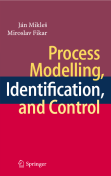## J. Mikleš, M. Fikar, Process Modelling, Identification, and Control. Springer Verlag, Berlin, 2007, ISBN 978-3-540-71969-4

Files to selected examples

Errata [PDF]

ChangeLog [TXT]

Process Modelling, Identification, and Control presents the time domain versus polynomial approach to modern process control. This book deals with:

• Process mathematical modelling (liquid storage, heat, and mass transfer models; models of chemical and biochemical reactors)
• State-space, and input-output process models (stability, BIBO stability, controllability, and observability of continuous-time systems; matrix fraction descriptions of MIMO systems)
• Dynamical process characteristics (time responses of linear systems; computer simulations; frequency characteristics of systems)
• Discrete-time process models (state-space, and input-output discrete-time process models; stability, controllability, and observability of discrete-time systems)
• Process identification (identification from step responses; recursive least squares methods)
• Design of simple controllers
• LQ, LQG, and H2 optimal control (optimal control and principle of minimum, dynamic programming; state observation, Kalman filter; state feedback with observer; polynomial interpretation of state feedback with observer; Youla-Kučera parametrisation, dual parametrisation; observer LQ control, state-space and polynomial interpretation; LQG control, state-space and polynomial interpretation; H2 optimal control, state-space and polynomial interpretation)
• Predictive control (optimal predictive control, predictor from state-space model, explicit approach, tuning)
• Adaptive control (discrete-time, and continuous-time self-tuning control; adaptive control of a tubular reactor)

The intended audience of this book includes graduate students but can also be of interest to practising engineers or applied scientists that are interested in modelling, identification, and process control. By using this book, control engineering students can obtain a knowledge of the analysis and design of control systems.

Review: "The book is well organized and presents the most important classical and modern essentials of control engineering. Suitable for senior under graduate and under graduate students as well as practical engineers and researchers interested in modeling and control process." (Seenith Sivasundaram, Zentralblatt MATH, Vol. 1121 (23), 2007)